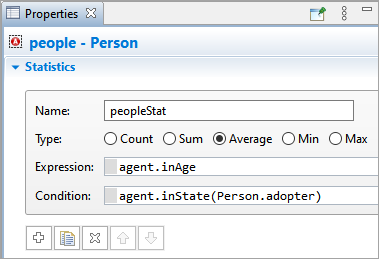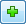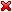AnyLogic

# Collecting statistics on agents

AnyLogic supports a convenient way of collecting statistics for population of agents.

There are several statistics types supported:

• Count. Counts agents satisfying the specified Condition.
• Sum. Iterates through all agents and evaluates the specified Expression for each agent. Returns the sum of obtained values.
• Average. Iterates through all agents and evaluates the specified Expression for each agent. Returns average of obtained values.
• Min. Iterates through all agents and evaluates the specified Expression for each agent. Returns the minimum obtained value.
• Max. Iterates through all agents and evaluates the specified Expression for each agent. Returns the maximum obtained value.

Sum, Average, Min, and Max can be calculated only for those agents, who meet the optional Condition. Say, we want to calculate the average age of product adopters in agent-based Bass Diffusion model. Let's assume we have people defined by agent type Person in this model. This type has a statechart with PotentialAdopter and Adopter states and age parameter defining the person's age. In this case our statistics should look like the following:To define new statistics function for a population of agents

1. Select the population element in the graphical editor or in the Projects view.
2. Open the Statistics section of the Properties view.
3. Click theAdd statistics button to create a new section defining one statistics field.4. Edit the name of the statistics function in the Name field. This name is used to identify and access this statistics function.
5. Choose the type of statistics function using the Type group of buttons.
6. Specify Expression and/or Condition using the controls below. While writing code in these fields, you can access an individual element of a population (an agent) as item.
7. To add more statistics fields, please repeat steps 3-6. If you do not need some statistics any more, you can remove the corresponding section by clicking thebutton.

The defined statistics field is accessed in the "function" style: by statistics Name followed with parenthesis, i.e. in the example described above, to calculate the defined statistics, call peopleStat() statistics function.

## Visualization of the agent population statistics

You can visualize the agent population statistics with charts.

You can study the technique in the given demo model. Here we have the people population with the NSusceptible(), NInfectious(), and NRecovered() statistics functions.

The collected statistics are visualized with the time plot. In the Data section of its properties, you can see the data items that correspond to the statistics functions. To visualize the statistics functions, you need to select the Value option and specify the name of the corresponding function in the Value field. Since the statistics functions belong to the population named people, here we use “people” as a prefix: people.NSusceptible(), people.NInfectious(), people.NRecovered().